## Characterization of Induction motor

##### Introduction

A 3Φ AC Induction motor can be mathematically modeled as an electrical and a mechanical system related by back-emf to speed and current-torque relationships. In this experiment, the electrical and mechanical parameters of the Induction motor are determined. These values are necessary to design proper control system to control motor speed, torque, or position.

##### Theoretical background

Motor model

Under steady-state operating condition, i.e. motor speed, magnitude and frequency of current and voltage, and torque are constants, the induction motor can be modeled (on a per-phase basis) as a transformer as shown below.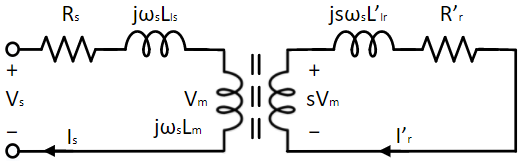On the left is the stator circuit and on the right is the rotor circuit. These are electrically isolated by the air-gap in between the stator and the rotor but are magnetically coupled. In a permanent magnet synchronous motor during steady-state, the rotor rotates at synchronous speed:

ωsync = 2P × ωs = 2P × 2πfs(1)

where, P is the number of poles and fs is the frequency of the applied voltage.

In case of an induction motor, the rotor rotates at a speed, slightly slower than the synchronous speed (under motoring mode):

ωmech = (1 - s) × ωsync(2)

where, s is the motor slip and is typically between 0.005 to 0.05.

The induced voltage on the rotor is directly proportional to the motor slip and the stator voltage across the magnetizing inductance. If the rotor was stationary, i.e. ωmech = 0, then slip s = 1 and the above circuit behave exactly like a transformer. When the rotor rotates at synchronous speed, i.e. ωmech = ωsync, then slip s = 0 and there is no voltage induced on the rotor because the rotor does not "see" any changing flux as it rotates at the same speed as the stator induced air-gap flux. For any speed in between, the induced rotor voltage magnitude is proportional to the slip and the frequency of the induced rotor voltage fr is given by:

fr = s × fs(3)

The equation of the rotor circuit is given in Eqn. 4. This can be modified by cancelling slip on both sides as done in Eqn. 5.

s × Vm = s × ωsLmI'r + R'rI'r(4)

Vm = ωsLmI'r + R'rsI'r(5)

This allows for further simplification of the equivalent circuit as shown below: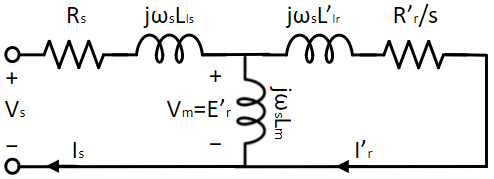The equivalent reflected rotor resistance R'rs, in the above equivalent circuit consists of two components namely, the reflected rotor resistance R'r and mechanical output equivalent resistance Rm = R'r1-ss. The power lost in Rm equals the output mechanical power given by:

Po = 32 × I2r,peak × R'r × 1 - ss = Tem × ωmech(6)

where, Tem is the motor electromagnetic torque and Ir,peak is the peak rotor current.

The equivalent circuit modified to reflect the mechanical model, given by Eqn. 7, is shown below:

Tem = Tc + Bωmech + Jmechdt(7)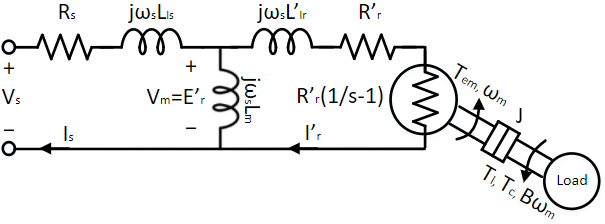where,

1. Vs : stator terminal phase voltage

2. Rs : per-phase stator resistance

3. Lls : per-phase stator leakage inductance

4. Lm : per-phase magnetizing inductance

5. R'r : per-phase rotor equivalent resistance

6. L'lr : per-phase rotor equivalent leakage inductance

7. s : rotor slip

8. J : rotor inertia

9. B : coefficient of viscous friction

10. Tc : torque due to Coulomb friction

11. Tem : output/electromagnetic torque

In the following section, the motor parameters are determined by further simplifying the circuit under different operating conditions.

Parameter estimation

In this experiment, the electrical and mechanical model parameters are determined as follows:

1. Rs: Use a multimeter. The resistance measured across two terminals is the line-line resistance. This must be divided by 2 to get per-phase resistance Rs. Alternatively, apply a constant voltage, Va of less than 2 V at the motor terminal and measure the steady-state stator current Is. Divide Vs by Is to obtain the line-line stator resistance, half of which give the stator phase resistance Rs.

2. Lm: Apply rated voltage Vs at rated frequency fs, at the motor terminals. At this condition, the motor should rotate with very low slip since there is no load torque other than the frictional component. Since the slip is small, the equivalent rotor resistance R'r/s is extremely large. Hence, the rotor can be considered at open circuited under no load condition. If the induction motor is coupled to a DC generator, more precise estimation can be done by running the generator in speed control with the reference speed set to synchronous speed. This essentially makes slip s = 0 and thus the rotor is open circuited. From the stator current and voltage, the sum of magnetizing inductance and stator leakage inductance, Ls = Lm + Lls, can be determined using the following equation:

R2s + (Ls × 2πfs)2 = V2s,peakI2s,peak(8)

In a practical induction motor, Lm >> Lls. Hence, the value obtained for Ls by solving the above equation can be reasonably approximated to be equal to Lm

3. R'r, Lls and L'lr: Lock the rotor so that it does not rotate. Apply 25% rated frequency and increase the applied voltage gradually, till the stator current reaches rated value. The reason for choosing 25% of rated frequency for this test is as follows. If a high enough frequency is applied, the impedance of the magnetizing path is much higher than that of the rotor, i.e. ωLm >> ωLls + R'r/s. Thus, the magnetizing branch impedance can be ignored for this test. But this frequency will not be reflective of the actual motor which under normal operation has rotor currents at frequency close to the rated slip frequency. Due to this the estimated resistance will be higher than the actual resistance due to skin-effect. If this test was done at very low frequency, close to slip frequency, then the magnetizing impedance is not large enough in comparison to rotor impedance to be ignored and this complicates the estimation of rotor parameters. Hence to maintain the simplicity of the former and the precision of the latter a frequency in between the rated stator frequency and the rated slip frequency is chosen, fs = 0.25 x frated. Under this condition, the equivalent circuit can be summarized as given by:

(Rs + R'r) + j × (Lls + L'lr) × 2πfs = VsIs(9)

Knowing the peak current Is,peak, peak voltage Vs,peak and the phase shift between the current and voltage Φ, the leakage inductance and rotor resistance can be estimated as follows:

R = Rs + R'r(10)

Ll = Lls + L'lr(11)

2πfs × LlR = tan(Φ)(12)

R2 + (Ll × 2πfs)2 = V2s,peakI2s,peak(13)

Solving these equations results in solution for R and Ll, from which the leakage inductances and rotor resistance can be determined using Eqn. 14 through 16. The last two equations are empirical relations.

R'r =R - Rs(14)

Lls = 25Ll(15)

Llr = 35Ll(16)

4. Tc and B: If the motor is not accelerating i.e. motor speed is a constant, and there is no load connected, the inertial torque component and load torque component in Eqn. 7 becomes zero, leading to the following equation:

Tem = Tc + Bωm(17)

Apply a 3Φ voltage of magnitude V and frequency f such that V/f = Vrated/frated, at the motor terminal. Measure the motor speed ωm, and stator peak current Is,peak. The rotor peak current can be computed from the stator peak current using current division as given by Eqn. 18. The electromagnetic torque Tem can be computed from the rotor peak current Ir,peak using Eqn. 6. Repeat the same for different voltages and plot the result, Tem (y-axis) vs. ωm (x-axis). The resulting plot will be linear. The slope of the plot equals the coefficient of viscous friction B and the y-axis intercept is the frictional torque Tc, due to Coulomb friction.

Ir,peak = Is,peak × 2πfs × Lm√((2πfs × (Lm + L'lr))2 + (R'rs)2)(18)

5. J: Apply a 3Φ voltage of magnitude V and frequency f such that V/f = Vrated/frated, at the motor terminal. Once the speed settles down, measure the speed ωm, and peak stator current Is,peak. At steady state, the electromagnetic torque Tem (Eqn. 7), solely compensates for drag due to friction. Disable the inverter so that the motor current dies-down to zero rapidly. After this, there is no electromagnetic torque and all the frictional losses must be supplied from the inertial energy. This leads to the motor to stop gradually. This is summarized in Eqn. 19, which is obtained by equating Tem and load torque Tl in Eqn. 7 to zero.

0 = Tfric + Jmdt(19)

At the moment the inverter is disabled, time t = tdis, the frictional torque component Tfric is same as, that of electromagnetic torque Tem(tdis) just before disabling the inverter, which can be computed from using Eqns. 6 and 18. Substituting this into Eqn. 11 yields:

J = Tem(tdis)mdt(tdis)(20)

Thus, the motor inertia can be obtained by dividing, the electromagnetic torque just before disabling the inverter, with the motor deceleration just after disabling the inverter.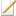Note

Disabling the inverter is not the same as setting the output voltage to 0. In the first case, the motor current extinguishes at a much faster rate than the rate at which the motor speed slows down. After this point, the inertial energy is not lost to armature resistance or fedback to the source. If the terminal voltage was set to zero, the inertial energy in addition to being lost in friction, will also be lost in the armature resistance because the back-emf will source the current due to the terminal being effectively shorted.

##### Real-time parameter estimationNote

For all computations in the steps below, the number of poles of the induction motor, P = 4.

1. Rs: Measure across the motor terminal using a multimeter. Divide by 2 to obtain per-phase resistance.

2. Lm:

1. Open Workbench and pin the Explorer and Properties dock.

2. Navigate to and open the IMMotor project file in Experiment2 folder usually in the following location: C:\Program Files (x86)\Sciamble\WorkBench v1\Examples\CUSPLab\AdvancedDrives.

3. Expand the project and open the IMElecEst model file (double clicking on the model node). Open the model properties (double click anywhere in the blank space of the open model). Copy the value in the property labelled Name, which if previously unchanged will be IMElecEst.

4. Since this project has more than one model file, the project must be notified as to which of these models should be run. To do this, open the project properties (double click on the project node in the Explorer dock) and paste the copied model name (IMElecEst) into the Start model/Function field.

5. Shown below is the IMElecEst model: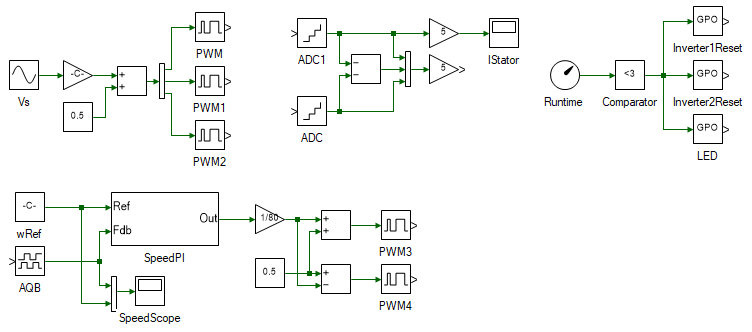The model consists of DC motor speed controller to maintain the motor at synchronous speed. On the top is 3Φ sine PWM, which controls the applied terminal voltage and frequency. To the right of it is 3Φ current measurement blocks. The phase A and C current are measured. The phase B current is computed by using the fact that the sum of the 3Φ current equals zero.

6. Set the DC motor reference speed to π * IMParam:frated in the Constant block labelled wRef.

To type the character π, click Alt+P. Pressing Alt will bring up the Greek keyboard.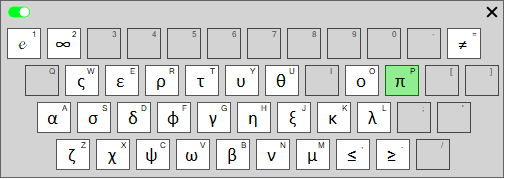7. Set the stator voltage Magnitude to IMParam:Vsrated and Frequency to IMParam:frated in the Sine block labelled Vs. These values are defined within IMParam script.

8. Turn ON the DC power supply and set the voltage to 40 V.

9. Ensure that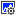button on the top dock, to transition to real-time mode, is pressed. Click the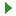button.

10. Open the scope named IStator to see the data log of phase-A stator current. After about 5 s, stop the data logging by clicking on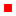button. Auto-focus the scope result by clicking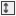in the Scope's toolbar.

11. Zoom in on the peak of the stator currents, once the motor has attained steady-state. Make note of this value.

12. Compute the value of Lm using Eqn. 8, where Vs,peak can be obtained from the IMParam script file's Vsrated value.

3. R'r, Lls and L'lr:

1. Since this is a blocked rotor test, set the DC motor reference speed to 0 in the Constant block labelled wRef.

2. Set the stator voltage Magnitude to IMParam:Vs and Frequency to IMParam:f in the Sine block labelled Vs. These values are defined within IMParam script.

3. Open the IMParam file within the project and set the variable f to 15 and Vs to 5.

4. Turn ON the DC power supply and set the voltage to 40 V.

5. Ensure thatbutton on the top dock, to transition to real-time mode, is pressed. Click the run button.

6. After about 5s turn off the DC power supply.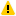Warning

Do not let the test run continuously for more than 10s. Since the stator current equals rated current or more, the motor tends to get heated quickly. In addition, the rotor is not rotating preventing the natural cooling offered by a rotating rotor. Due to this, make sure to turn of the DC power supply after every reading and turn it back on only when needed.

7. Open the stator phase-A current scope and look at the peak stator current at stead-state. In the current is less than rated current of 2.5A, increment the induction motor stator voltage V in IMParam script by small steps (of 2V).

If the peak current is greater than the rated current, reduce the voltage by large steps (of 5V) to bring the current to within rated current limit.

Keep repeating this till the peak current converges close to the rated current.

8. Ensure that the DC power supply is turned off.

9. Zoom in on the peak of the stator current, once the motor has attained steady-state. Make note of this value.

10. Zoom in on the zero-crossing (negative to positive transition) of the stator current, once the motor has attained steady-state. Make note of the time tzc at which the zero-crossing occurs. The number of stator voltage cycle between time t = 0 and t = tzc is given by:

N = floor((tzc × fs))(21)

where, f is the frequency of the applied voltage and floor is the value rounded to the smallest of the two closest integers.

From this the phase shift between the stator current and voltage can be computed by:

Φ = 2π × (tzc × fs - N)(22)

11. Compute the value of R'r, Lls and L'lr from phase shift and the peak stator current, using Eqns. 10 through 16.

12. Close the IMElecEst model.

4. Tc and B:

All three parameters are estimated using the same method, in this step.

1. Open the IMBEst model within the same project.

2. Open the model properties and copy the property, Name. Go to project properties and set the Start model/Function to the copied value.

3. Set the stator voltage Magnitude to IMParam:Vs and Frequency to IMParam:f in the Sine block labelled Vs. These values are defined within IMParam script.

4. Open the IMParam file within the project and set the variable f to 15 and Vs to f * Vsrated / frated.

5. Ensure thatbutton on the top dock, to transition to real-time mode, is pressed. Click the run button.

6. After about 5s turn off the DC power supply.

7. Make note of the results in a table of following layout:

Vs,peak (V) Is,peak (A) ωmech (rads/s) Ir,peak (A) Po (W) Tem (Nm)
`        `
`        `
`        `
`        `
`        `
`        `

where, Vs,peak is the stator peak phase voltage which can be obtained from the Magnitude property of Sine block labelled Vs, Is,peak is the peak stator current logged in Scope IStator, and ωmech is the motor speed logged in Scope wmech. All these values must be measured after reaching steady-state after applying the desired voltage. The remaining table entries are computed based on these values using Eqns. 6 and 18.

8. Repeat the previous three steps for different motor frequency f, from 15 Hz to 45 Hz, in steps of 3 Hz. The voltage Vs in the IMParam script must always be maintained such that V/f = Vrated/frated.

9. Turn OFF the DC power supply and unplug the USB cable.

10. Plot the result of Tem (y-axis) vs ωmech (x-axis).

Measure the slope of the get an estimate of coefficient of viscous friction B and the y-axis intercept to get the frictional torque due to Coulomb friction Tc.

5. J:

1. Open the IMJEst model within the same project.

2. Open the model properties and copy the property, Name. Go to project properties and set the Start model/Function to the copied value.

3. The model is shown below. The stator voltage is set to motor rated voltage IMParam:Vsrated and rated frequency IMParam:frated. When the motor has reached its steady-state, the inverter is disabled at t = 3 s. This puts the inverter terminals at high-impedance so that, the inertial energy is lost only in the frictional component.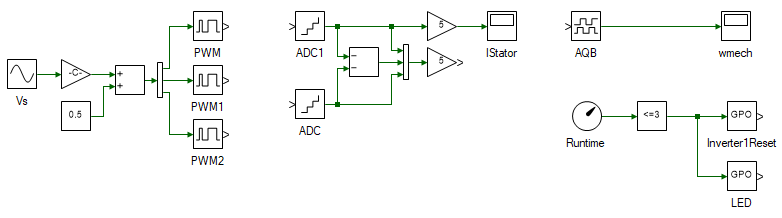4. Re-connect the USB and turn on the DC power supply.

5. Ensure thatis pressed. Clickto run the model in real-time.

6. Open the scope wmech to observe the motor speed. When the data has been logged for 5 s, click theto stop the data logging. The results in will look something similar: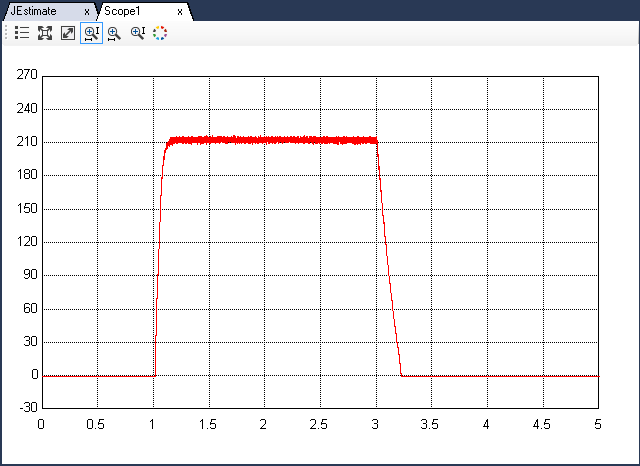7. Zoom in on the speed at t = 3s and determine the slope as shown below.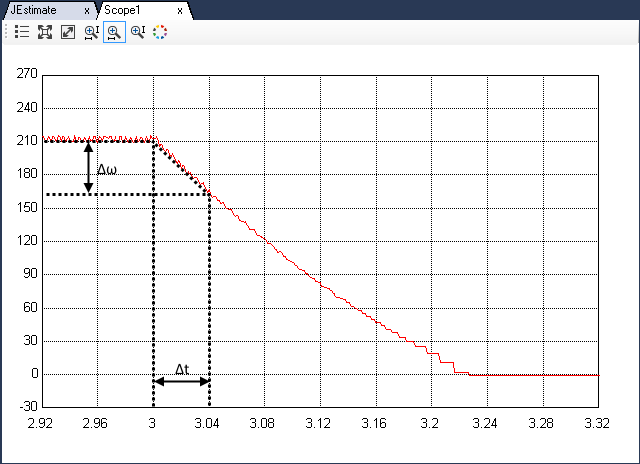8. In the stator current plot, make note of the current's peak magnitude, before the inverter was disabled at t = 3 s. Using this current, speed and speed slope, the inertia is calculated using Eqns. 6, 18 and 20.

6. Turn OFF the DC power supply and disconnect all the connections including the USB.

This concludes the experiment on DC motor characterization. The parameters estimated in this experiment will be used in the following experiments, to tune a PI loop to control the motor speed.

##### Lab report and reading assignment
1. Attach a plot of Is from Lm estimation.

2. Attach a plot of Is from R'r estimation.

3. Attach plots of Is and ωmech for different f from B estimation.

4. Attach plot of Is and ωmech from J estimation.

5. Attach the table from B estimation.

6. List the results of all final parameter values.

7. Using these parameters design an Induction motor abc model and show motor current and speed for an applied voltage of 14 V (ll-rms) at 50 Hz.

< Introduction : previous topic